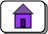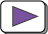created: 05/29/2009; revised 05/24/2015, 04/23/23# Appendix H — Converting Decimal Representation to Binary

This chapter shows how to find the binary representation of an integer, assuming that you start with its decimal representation. You should already have read chapters one through eight.

#### Chapter Topics:

• Repeated Division Method
• Uses repeated division by two.
• Bits are produced least significant bits first.
• Same method as used in chapter 7.
• Repeated Subtraction Method
• Done by repeated subtraction of the best-fitting power of two.
• Start with the largest power of two that fits into the integer.
• Bits are produced most significant bits first.

Let us start by looking at the Repeated Division Method.

### QUESTION 1:

If you divide a positive integer by two (using integer division) what are the possible values for the remainder?

Hint: try dividing 4 by two.
try dividing 5 by two.
try dividing 6 by two.